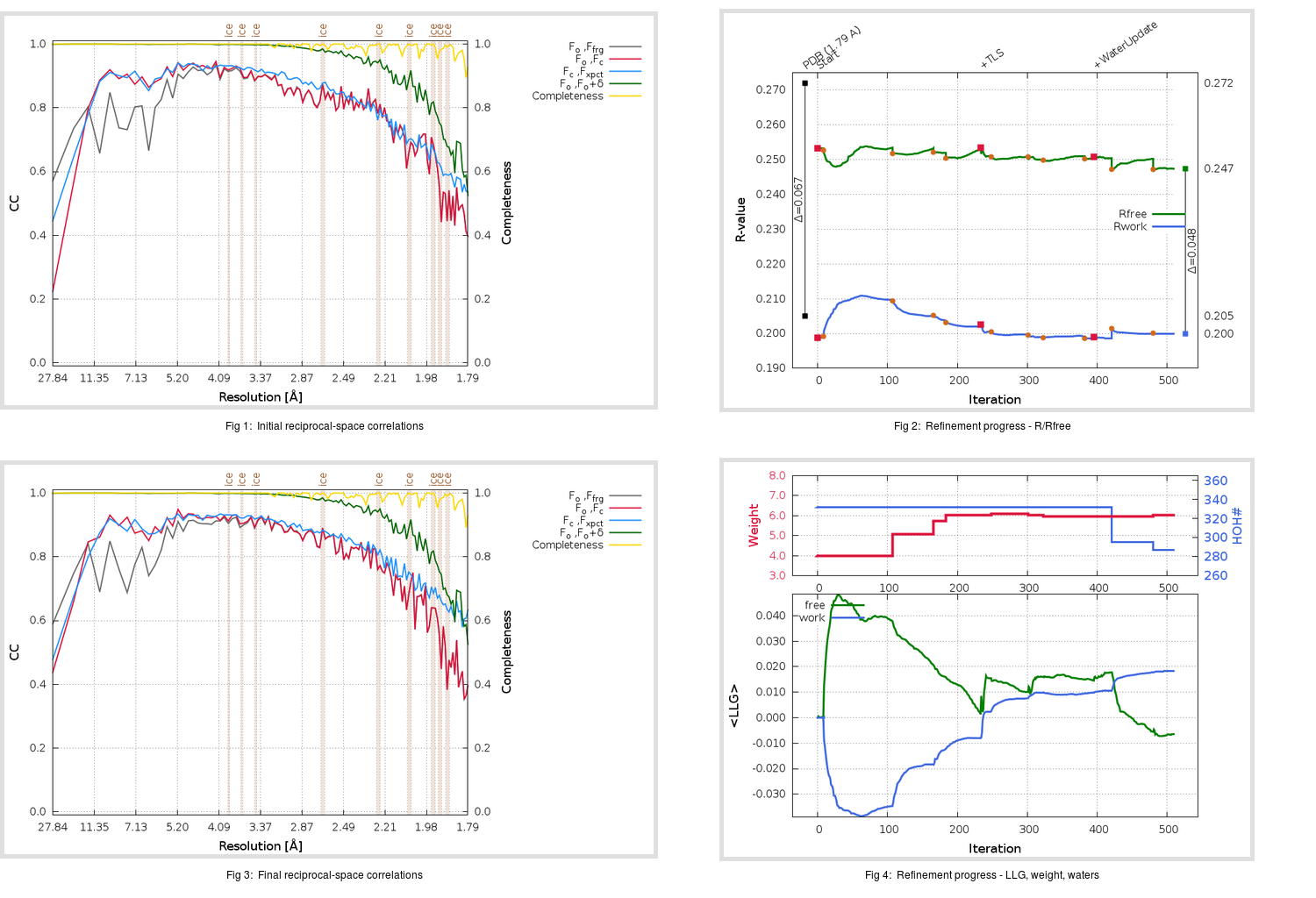Content:

```    Diffraction limits & principal axes of ellipsoid fitted to diffraction cut-off surface:
1.795         0.8964   0.0000   0.4433       0.995 a* + 0.101 c*
1.844         0.0000   1.0000   0.0000       b*
1.698        -0.4433   0.0000   0.8964      -0.758 a* + 0.653 c*
```

## Deposited

` `
 Date deposited Date data collection Resolution R, Rfree 20200315 20200305 1.79 0.2020 0.2720

Molprobity (CCP4 7.0 version) summary:

```Ramachandran outliers =   0.33 %
favored =  97.02 %
Rotamer outliers      =   1.52 %
C-beta deviations     =     0
Clashscore            =   6.76
RMS(bonds)            =   0.0132
RMS(angles)           =   1.80
MolProbity score      =   1.68
Resolution            =   1.79
R-work                =   0.2020
R-free                =   0.2720
```

```Number of waters      =   332

<B> (all atoms) =   32.10 ( sd =   10.30 ) for       2727 non-hydrogen atoms
<B>   (protein) =   30.74 ( sd =    9.70 ) for       2370 non-hydrogen atoms
<B>     (water) =   40.88 ( sd =    9.71 ) for        332 non-hydrogen atoms
<B>    (others) =   44.85 ( sd =   11.12 ) for         25 non-hydrogen atoms

B min/max       (all non-hydrogen atoms) =   16.78 /   81.68
B min/max   (protein non-hydrogen atoms) =   16.78 /   81.68
B min/max     (water non-hydrogen atoms) =   17.18 /   74.00
B min/max     (other non-hydrogen atoms) =   30.94 /   57.05
```

## BUSTER (re-)refinement

` `

Molprobity (CCP4 7.0 version) summary:

```Ramachandran outliers =   0.33 %
favored =  98.34 %
Rotamer outliers      =   1.52 %
C-beta deviations     =     0
Clashscore            =   2.54
RMS(bonds)            =   0.0118
RMS(angles)           =   1.57
MolProbity score      =   1.18
Resolution            =   1.79
R-work                =   0.1999
R-free                =   0.2474
```

```Number of waters      =   287

<B> (all atoms) =   30.25 ( sd =    8.90 ) for       2682 non-hydrogen atoms
<B>   (protein) =   28.89 ( sd =    7.90 ) for       2370 non-hydrogen atoms
<B>     (water) =   40.40 ( sd =    9.47 ) for        287 non-hydrogen atoms
<B>    (others) =   42.13 ( sd =   11.00 ) for         25 non-hydrogen atoms

B min/max       (all non-hydrogen atoms) =   15.39 /   68.57
B min/max   (protein non-hydrogen atoms) =   15.39 /   65.74
B min/max     (water non-hydrogen atoms) =   16.51 /   68.57
B min/max     (other non-hydrogen atoms) =   31.77 /   53.82
```

Refinement progression:Results:

` `
 File Remark 5REZ_aB_refine.01_03_refine.pdb.gz exact refinement commands are in header 5REZ_aB_refine.01_03_refine.mtz.gz including original deposited data and several re-refinement map coefficients 5REZ_aB_refine.01_03_BUSTER_model.cif.gz including any non-standard compound restraints 5REZ_aB_refine.01_03_BUSTER_refln.cif.gz